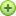# New to QlikView

Discussion board where members can get started with QlikView.

Announcements
Modernize Your QlikView Deployment webinar, Nov. 3rd. REGISTER
cancel
Showing results for
Did you mean:
HighlightedMaster II

## Colors based on salaries

Hi All,

I want to show the colors based on the Salaries of the Employees.

If EMP SAL>=4500 show green,EMP SAL>=3500 show ,EMP SAL<=2500 show red.

1 Solution

Accepted Solutions
HighlightedSpecialist

use this expression in expression background or text colors

=If(sum(SAL)>=4500,Green(),If(sum(SAL)>=3500,LightGreen(),If(sum(SAL)<=2500,Red(),LightCyan())))10 Replies
HighlightedSpecialist

use this expression in expression background or text colors

=If(sum(SAL)>=4500,Green(),If(sum(SAL)>=3500,LightGreen(),If(sum(SAL)<=2500,Red(),LightCyan())))HighlightedMVP

Cannot open your qvw because I am using PE version of QlikView, but may be this for your background color expression:

If([EMP SAL]<= 2500, Red(),

If([EMP SAL]>= 3500 and [EMP SAL] < 4500, Yellow(),

If([EMP SAL]>= 4500, Green())))

Note: You don't have any color for between 2500 and 3500.

HTH

Best,

Sunny

HighlightedNot applicable

Hi ,

In the background color of expression write this expression for color:

if(sum(SAL)>=4500 ,green(),

if((sum(SAL)>=3500 and  sum(SAL)<4500),yellow(),

if(sum(SAL)<=2500,red())))

Thanks

Khushboo

HighlightedSpecialist

hi!

1. Name the expression Salary.

2. Dimenstion ->->Text Color

=if([Salary]>4500,green(),

if([Salary]>3500, yellow(),

if([Salary]<=2500, red()))

)

HighlightedPartner

In the color property of table you can set the color amount lower than,upper than,and average  whatever condition you want...

HighlightedSpecialist

Hi

=if(sum(SAL)>=4500,Green(),if(sum(SAL)>=3500 and sum(SAL) <4500 ,Yellow(),if(sum(SAL)<=2500,Red())))

HighlightedSpecialist II

Hi,

May be this?? also find sample app.HighlightedMVP & Luminary

Hi,

Try this background color expression

If([EMP SAL]>=4500, Green(),

If([EMP SAL]>=3500, Blue(),

If([EMP SAL]<=2500, Red())))

Hope this helps you.

Regards,

Jagan.

HighlightedNot applicable

Hi,

See the attached qvw.

the expretion like this in expretions tab click onsymbal and in text colet write down the expretion as bellow.

=IF(sum(SAL)<=2500,Red(),

IF(sum(SAL)>=3500,Green(),

IF(sum(SAL)>=4500,Yellow(),LightBlue())))